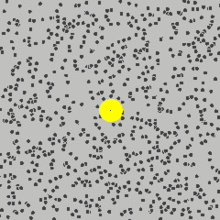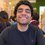# Question: Is Brownian Motion truly "random"?

Upon reading the very well written wiki of the day concerning Albert Einstein, the paragraph concerning Brownian motion caught my eye. Especially the sentence, "Brownian motion is the random motion of small object (such as a speck of pollen) while suspended in a fluid (such as water). Einstein demonstrated that this motion was the result of many tiny molecules colliding with the pollen".

Out of curiosity, is Brownian motion truly "random"? The electrical force exerted from one particle to another can be calculated (for example, using $\overset { \rightarrow }{ { F }_{ e } } ={ k }_{ e }\dfrac { { q }_{ 1 }{ q }_{ 2 } }{ { r }^{ 2 } } \overset { \wedge }{ r }$ where ${ k }_{ e }=\dfrac { 1 }{ 4\pi { \epsilon }_{ 0 } }$), and in turn, given enough computational power; every force every particle exerts on an objects can be calculated. So why would Brownian motion be said to be random?Note by Andrew Tawfeek
5 years, 4 months ago

This discussion board is a place to discuss our Daily Challenges and the math and science related to those challenges. Explanations are more than just a solution — they should explain the steps and thinking strategies that you used to obtain the solution. Comments should further the discussion of math and science.

When posting on Brilliant:

• Use the emojis to react to an explanation, whether you're congratulating a job well done , or just really confused .
• Ask specific questions about the challenge or the steps in somebody's explanation. Well-posed questions can add a lot to the discussion, but posting "I don't understand!" doesn't help anyone.
• Try to contribute something new to the discussion, whether it is an extension, generalization or other idea related to the challenge.

MarkdownAppears as
*italics* or _italics_ italics
**bold** or __bold__ bold
- bulleted- list
• bulleted
• list
1. numbered2. list
1. numbered
2. list
Note: you must add a full line of space before and after lists for them to show up correctly
paragraph 1paragraph 2

paragraph 1

paragraph 2

[example link](https://brilliant.org)example link
> This is a quote
This is a quote
    # I indented these lines
# 4 spaces, and now they show
# up as a code block.

print "hello world"
# I indented these lines
# 4 spaces, and now they show
# up as a code block.

print "hello world"
MathAppears as
Remember to wrap math in $$ ... $$ or $ ... $ to ensure proper formatting.
2 \times 3 $2 \times 3$
2^{34} $2^{34}$
a_{i-1} $a_{i-1}$
\frac{2}{3} $\frac{2}{3}$
\sqrt{2} $\sqrt{2}$
\sum_{i=1}^3 $\sum_{i=1}^3$
\sin \theta $\sin \theta$
\boxed{123} $\boxed{123}$

Sort by:

The answer to your questions depends on what you consider to be truly random. If you consider something that you can't predict truly random, then yes, Brownian motion can be considered truly random.

Having said that, most people, especially in science circles don't find the premise 'things one can't predict' sufficient to define something as truly random. I would argue that Brownian motion is not truly random, because if you were able to measure the initial positions and velocity vectors of all the particles involved, you could calculate the outcome of your Brownian movement. The problem is that you don't usually know all the initial conditions and therefore can only treat the problem as if it was truly random. That is why the most common way of simulating Brownian motion is with random walks.

- 5 years, 4 months ago

Ohh, I see. Thank you for your response!

I likewise thought that it shouldn't be considered random considering it technically is possible (theoretically for now) to find and calculate everything needed to trace the exact motion an object would undergo. I'm glad to see I wasn't alone on this. :)

Although, prior to your response I did have doubts in this method of thinking. I thought perhaps it is called random because it wouldn't be possible to measure all the forces due to some possible quantum mechanics interferences with the measuring process. Would you imagine that QM could possibly play a role in Brownian motion?

- 5 years, 4 months ago

It has a lot to do with QM.

In Feynman's space-time approach to nonrelativistic quantum mechanics, the wave function may be considered to be the sum of path integrals over Brownian-motion trajectories. This formalism is entirely equivalent to the Schrödinger-equation treatment with its intrinsic indeterminacy. Pursuing the analogy with Brownian motion further, one may introduce a hidden variable corresponding to particle velocity in order to represent the wave function as a sum of path integrals taken over phase-space trajectories.

- 5 years, 4 months ago

So this would correspond to a reforging of quantum mechanics, expressing the wave function in terms of random walks in some variables which gives the same result as the probabilistic consideration of Planck's original QM? That's news to me.

- 5 years, 4 months ago

That surprised me too.

Have a look at this.

http://www.feynmanlectures.caltech.edu/I_41.html

- 5 years, 4 months ago

So basically, you have no idea what you're saying and just lifted that paragraph from somewhere, and you won't bother to explain to me what you mean.

- 5 years, 4 months ago

Well I am no expert in this field, I am still a student! I found that content useful, so I put it up for eveyone to read.

- 5 years, 4 months ago

@Jake Lai @David Mattingly I'd really like to hear your opinions on this (:

- 5 years, 4 months ago

I'm not as great as you think.

Anyway, "randomness" can perhaps be attributed to ignorance. Random walks seem to model Brownian motion very well, so we use them. We just create models in science, see if they works, and then try to give them some physical interpretation after the fact, if at all possible.

- 5 years, 4 months ago

Use $\hat{r}$ (\hat{r}) for unit vectors.

- 5 years, 4 months ago

That coulomb law is only appicable to the expectation values of position operator if you're dealing with precision (QM)... So, on average that is true...but actually it is truly random...though defining "random" is really difficult.

- 5 years, 4 months ago

Yes, it is.

- 5 years, 4 months ago